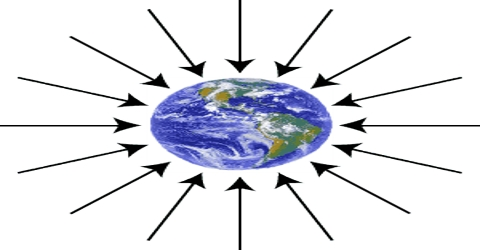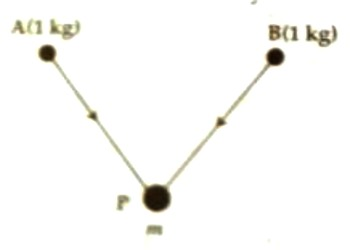# Gravitational Field Intensity or Strength

Gravitational field Intensity or Strength

Definition: The gravitational field intensity or strength of an object at any point in the gravitational field is defined as the force experienced by a unit mass placed at that point. So, Gravitational field intensity means if we bring a unit test mass from infinity to a gravitational field, then the gravitational force acted on that unit test mass due to a comparable bigger mass for which the gravitational field is created, is called gravitational field intensity.

For a non-contact force, the source mass and test mass interact with each other by means of a gravitational field. If the force acting on a body of mass m placed at a point in the gravitational field is F, then gravitational field intensity at that point is –

g or E = F/m … … … (1)It is seen from this equation that as the value of m increases E decreases. It is a vector quantity. It has both magnitude and direction. If more than one field intensity is acting at any point, then the resultant field intensity is obtained by vector addition of individual intensity. The direction of the field intensity indicates the direction of the field. Sometimes, an only gravitational field is written instead of gravitational field intensity. In S. I. system unit of intensity is Nkg-1.In order to understand the direction of gravitational intensity let a body of mass m be placed at a point P [Figure]. For determining gravitational field intensity of that body at point A let us assume that a body of unit mass is present at that point. Now the unit mass placed at point A will experience attractive force along with PA. So, the direction of the intensity at point A will be along with AP. Similarly, the direction of intensity at point B will be along with BP. Hence according to equation (1), field intensity in vector form can be written as,

EG = F/m

and in that case force, F= EG x m

Now we will see what is the meaning of intensity 4 Nkg-1 at a point in the gravitational field? In answer, we can say that if at any point in the gravitational field 1 kg mass is placed it will experience a force of 4 N or if 4 kg mass is placed in that field it will experience a force of 1 N.

Gravity is a good example – we know there is the acceleration due to gravity of about 9.8 m/s2 down at every point in the room. Another way of saying this is that the magnitude of the Earth’s gravitational field is 9.8 m/s2 down at all points in this room.

On the earth’s surface at the poles, we find gravity will be the strongest. The earth is an oblate spheroid, and that means it bulges out in the middle (the equator). That also means the poles end up a little closer to the center of gravity. That is why on the surface of the earth, at the poles the intensity of gravity is the maximum.